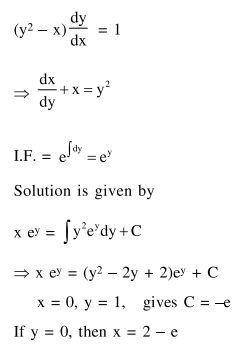# Let y=y(x) be the solution curve of theQuestion:

Let $y=y(x)$ be the solution curve of the

differential cquation, $\quad\left(\mathrm{y}^{2}-\mathrm{x}\right) \frac{\mathrm{dy}}{\mathrm{dx}}=1$,

satisfying $\mathrm{y}(0)=1$. This curve intersects the $x$-axis at a point whose abscissa is :

1. $2+\mathrm{e}$

2. 2

3. $2-\mathrm{e}$

4. $-\mathrm{e}$

Correct Option: , 3

Solution: